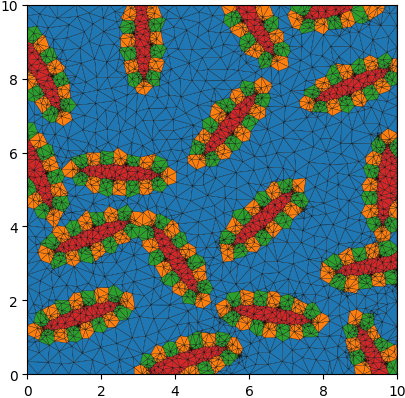# Grain Neighborhoods¶

## Python Script¶

The basename for this file is `grain_neighborhoods.py`. The file can be run using this command:

```microstructpy --demo=grain_neighborhoods.py
```

The full text of the script is:

```from __future__ import division

import os

import numpy as np
import scipy.integrate
import scipy.stats
from matplotlib import pyplot as plt

import microstructpy as msp

# Define the domain
domain = msp.geometry.Square(corner=(0, 0), side_length=10)

# Define the material phases
a_dist = scipy.stats.lognorm(s=1, scale=0.1)
matrix_phase = {'fraction': 1,
'material_type': 'matrix',
'shape': 'circle',
'area': a_dist}

neighborhood_phase = {'fraction': 1,
'material_type': 'solid',
'shape': 'ellipse',
'a': 1.5,
'b': 0.6,
'angle_deg': scipy.stats.uniform(0, 360)}

phases = [matrix_phase, neighborhood_phase]

# Create the seed list
seeds = msp.seeding.SeedList.from_info(phases, domain.area)
seeds.position(domain)

# Replace the neighborhood phase with materials
a = neighborhood_phase['a']
b = neighborhood_phase['b']
r = b / 3
n = 16

t_perim = np.linspace(0, 2 * np.pi, 201)
x_perim = (a - r) * np.cos(t_perim)
y_perim = (b - r) * np.sin(t_perim)
dx = np.insert(np.diff(x_perim), 0, 0)
dy = np.insert(np.diff(y_perim), 0, 0)
ds = np.sqrt(dx * dx + dy * dy)
arc_len = scipy.integrate.cumtrapz(ds, x=t_perim, initial=0)
eq_spaced = arc_len[-1] * np.arange(n) / n
x_pts = np.interp(eq_spaced, arc_len, x_perim)
y_pts = np.interp(eq_spaced, arc_len, y_perim)

repl_seeds = msp.seeding.SeedList()
geom = {'a': a - 2 * r, 'b': b - 2 * r}
for sn, seed in enumerate(seeds):
if seed.phase == 0:
repl_seeds.append(seed)
else:
center = seed.position

geom['center'] = center
core_seed = msp.seeding.Seed.factory('ellipse', phase=3,
position=seed.position, **geom)
repl_seeds.append(core_seed)

x_ring = center + x_pts * np.cos(theta) - y_pts * np.sin(theta)
y_ring = center + x_pts * np.sin(theta) + y_pts * np.cos(theta)
for i in range(n):
phase = 1 + (i % 2)
center = (x_ring[i], y_ring[i])
ring_geom = {'center': center, 'r': r}
ring_seed = msp.seeding.Seed.factory('circle', position=center,
phase=phase, **ring_geom)
if domain.within(center):
repl_seeds.append(ring_seed)

# Create polygon and triangle meshes
pmesh = msp.meshing.PolyMesh.from_seeds(repl_seeds, domain)
phases = [{'material_type': 'solid'} for i in range(4)]
phases['material_type'] = 'matrix'
tmesh = msp.meshing.TriMesh.from_polymesh(pmesh, phases, min_angle=20,
max_volume=0.1)

# Plot triangle mesh
colors = ['C' + str(repl_seeds[att].phase) for att in tmesh.element_attributes]
tmesh.plot(facecolors=colors, edgecolors='k', linewidth=0.2)

plt.axis('square')
plt.xlim(domain.limits)
plt.ylim(domain.limits)

file_dir = os.path.dirname(os.path.realpath(__file__))
filename = os.path.join(file_dir, 'grain_neighborhoods/trimesh.png')
dirs = os.path.dirname(filename)
if not os.path.exists(dirs):
os.makedirs(dirs)
```

## Domain¶

The domain of the microstructure is a `microstructpy.geometry.Rectangle`, with the bottom left corner at the origin and side lengths of 8 and 12.

## Phases¶

There are initially two phases: a matrix phase and a neighborhood phase. The neighborhood phase will be broken down into materials later. The matrix phase occupies two thirds of the domain, while the neighborhoods occupy one third.

## Seeds¶

The seeds are generated to fill 1.1x the area of the domain, to account for overlap with the boundaries. They are positioned according to random uniform distributions.

## Neighborhood Replacement¶

The neighborhood seeds are replaced by a set of three different materials. One material occupies the center of the neighborhood, while the other two alternate in a ring around the center.

## Polygon and Triangle Meshing¶

The seeds are converted into a triangular mesh by first constructing a polygon mesh. Each material is solid, except for the first which is designated as a matrix phase. Mesh quality controls are specified to prevent high aspect ratio triangles.

## Plotting¶

The triangular mesh is plotted and saved to a file. Each triangle is colored based on its material phase, using the standard matplotlib colors: C0, C1, C2, etc. The output PNG file is shown in Fig. 24.Fig. 24 Triangular mesh of microstructure with seed neighborhoods.¶